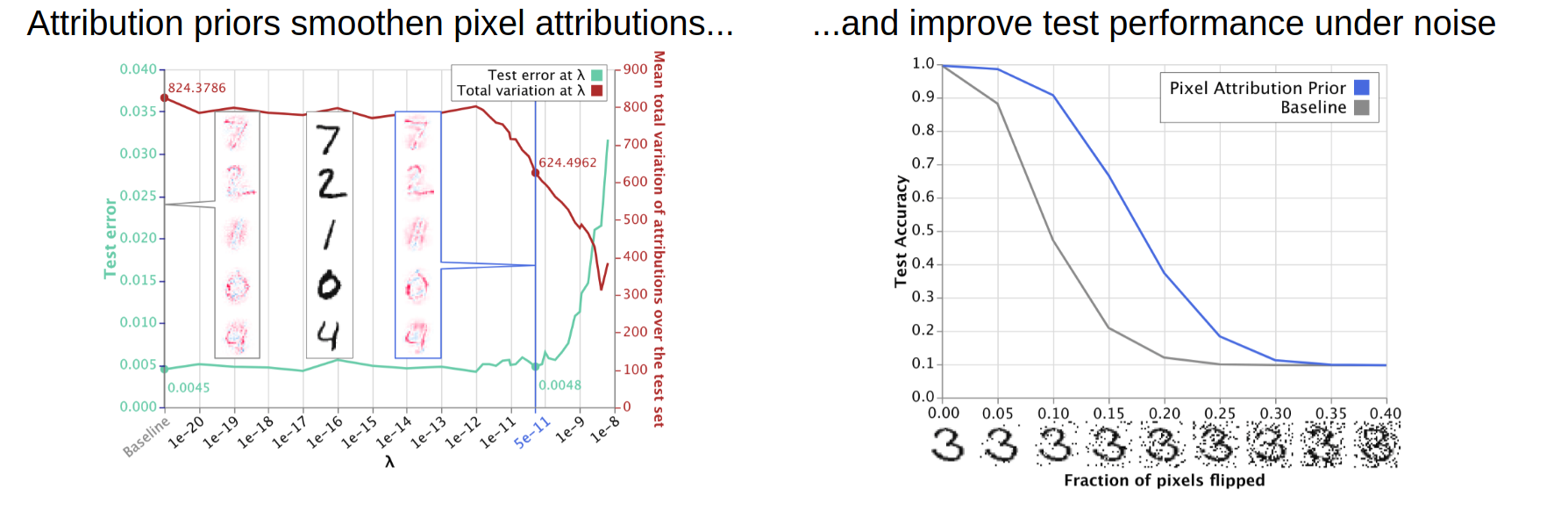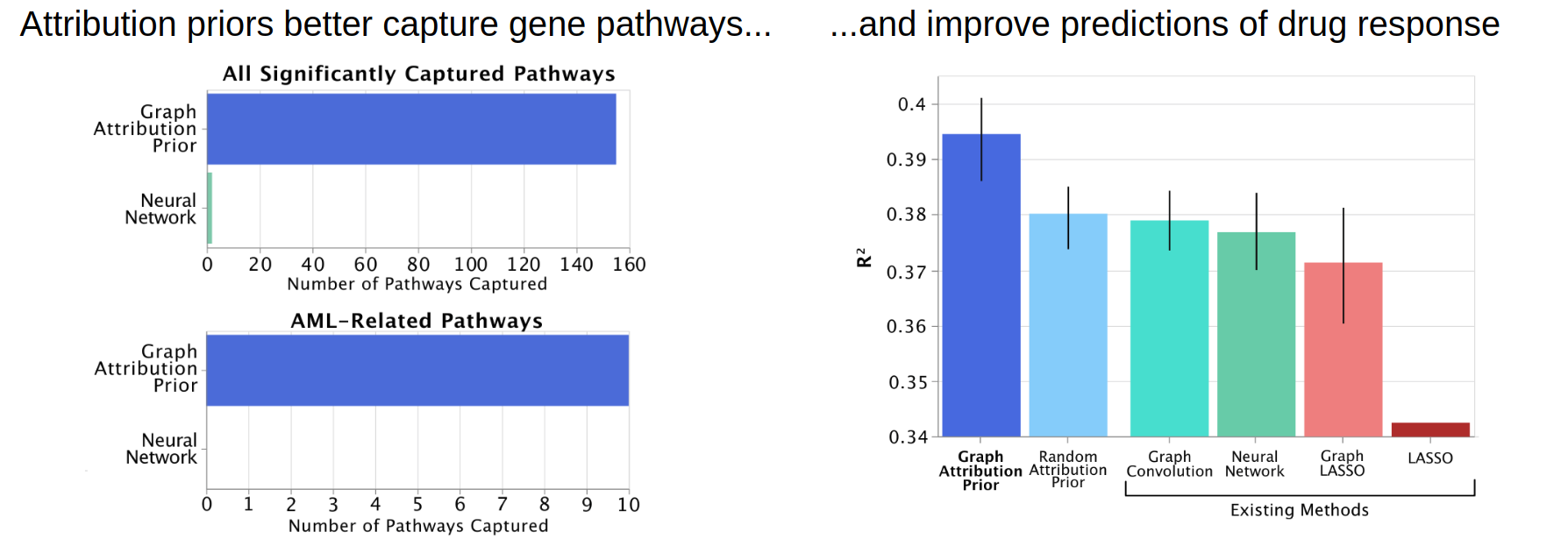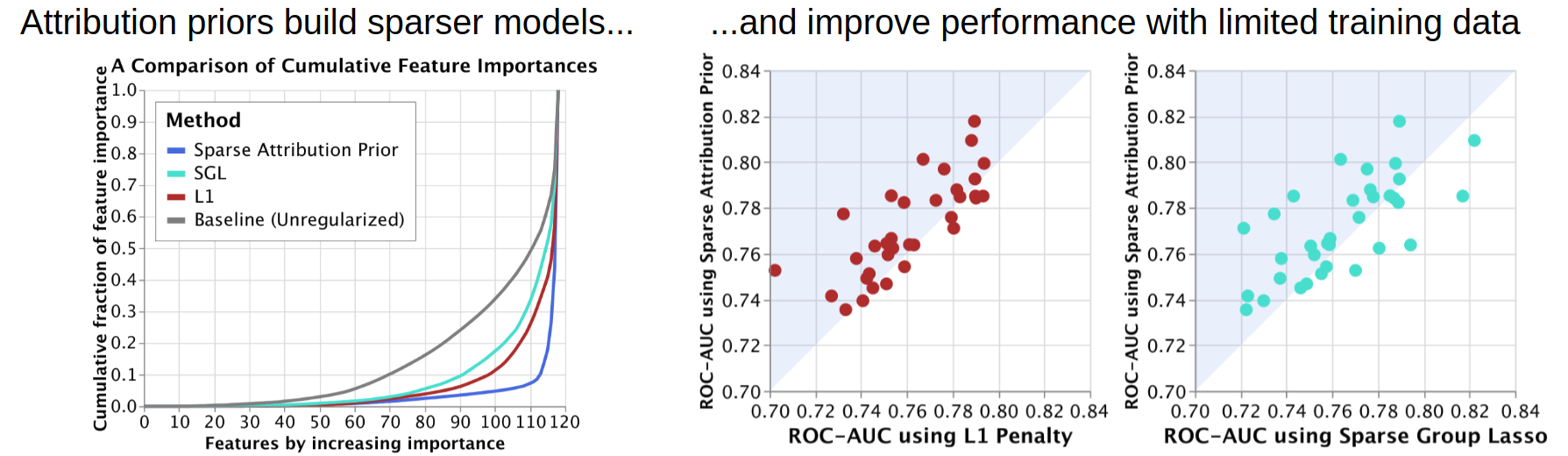## Attribution Priors

A repository for training explainable models using attribution priors.

This repository contains tools for connecting the machine learning topics of model priors and model explanations with a new method called attribution priors, discussed in our paper "Learning Explainable Models Using Attribution Priors". This package contains:

• A differentiable axiomatic feature attribution method called expected gradients.
• Tensorflow operations to directly regularize expected gradients attributions during training.
• Examples of how arbitrary differentiable functions of expected gradient attributions can be regularized during training to encode prior knowledge about a modeling task.

For more guidance about how to use this repository/how to train with attribution priors, see the `example_usage.ipynb` notebook
in the top level directory of this repository, and the Installation and Usage Section of this README.

## Examples

So what exactly are attribution priors and why would you want to use them? The examples here provide three ways in which
you can use attribution priors to improve network performance and interpretability. We use these examples
in our paper. However, attribution priors are not limited to the examples here.

### Image Data (mnist)

In the `mnist` folder, we give examples about how to train models that have smoother attributions over pixels, which in turn
leads to better performance on noisy test data. Click through the notebooks in that folder to see more.### Gene Expression Data (graph)

In the `graph` folder, the notebook shows how penalizing differences between the attributions of neighbors in an arbitrary graph
connecting the features can be used to incorporate prior biological knowledge about the relationships between genes,
yield more biologically plausible explanations of drug response predictions, and improve test error.### Tabular Data (sparsity)

In the `sparsity` folder, the notebook shows how encouraging inequality in the distribution of feature attributions
can build sparser models that can perform more accurately when training data is limited.## Installation

The easiest way to install this package is by using pip:

``````pip install attributionpriors
``````

Alternatively, you can clone this repository directly to explore and re-run the examples provided here.

## Compatability

The code in this repository was written to support TensorFlow versions r1.8 and up, and works with both Python 2 and 3. Although we plan to support the Keras API in the future,
only Keras models that directly use TensorFlow sessions to train are supported.

## Usage

This package provides a simple API that can be used to define attribution priors over neural networks that can be dropped in to existing TensorFlow code.
In order to train using our implementation of attribution priors in your own code, you need to follow the following four steps:

### 1: Importing

``````#Other import statements...
from attributionpriors.ops import AttributionPriorExplainer
``````

### 2: Wrapping your input tensor using an AttributionPriorExplainer object

Where normally you would write code like this:

``````input_op = get_input() #Returns some tensor of shape [batch_size, feature_dim0, feature_dim1, ...] that you feed into a TensorFlow model
``````

Add the following lines in green:

``````+ explainer = AttributionPriorExplainer()
input_op = get_input()
+ input_op, train_eg = explainer.input_to_samples_delta(input_op)
``````

`input_op` can be a placeholder, a constant, or any other TensorFlow tensor that you use to feed data, like images or feature vectors, into a model.
The above code modifications create operations that alternate between feeding your model normal input and input interpolated between a sample and a reference.
This is used to compute expected gradients.

### 3: Defining the Expected Gradients Tensor

The code for getting your expected gradients tensor depends on whether your model outputs a single number (e.g. in regression),
or multiple (e.g. in classification tasks).

#### Single-output Tasks (like regression)

If your code to define your model normally looks like this:

``````output_op = model(input_op) #output_op is a [batch_size] shaped tensor of floats
``````

Add the following line in green:

``````  output_op = model(input_op)
+ expected_grads_op = explainer.shap_value_op(y_pred, cond_input_op)
``````

#### Multi-output Tasks (like classification)

If instead, you are predicting multiple classes, you need to define which class you want to take expected gradients with respect to.
Although our repository supports taking expected gradients with respect to ALL output classes (simply by using the code above in the single-output tasks),
it is more computationally efficient and more intuitive to take expected gradients with respect to the true class. That is, if you have an image of, say, a car,
you should take attributions with respect to the car output class. Our repository supports this as follows:

``````label_op  = get_labels() #label_op should be a [batch_size] shaped tensor of integers specifying which class each input in input_op belongs to
output_op = model(input_op) #output_op is a [batch_size] shaped tensor of floats
+ expected_grads_op = explainer.shap_value_op(y_pred, cond_input_op, label_op)
``````

This will return a tensor operation that represents attributions with respect to the true class of each example.

### 4: Training with an Attribution Prior

First, you need to define a loss function with respect to the `expected_grads_op` tensor. You can look to the examples section of the README for some examples
of attribution priors, or you can read our original paper. For example, we use the total variation loss for images to get models with smoother explanations:

``````eg_loss_op  = tf.reduce_mean(tf.image.total_variation(expected_grads_op))
eg_train_op = tf.train.AdamOptimizer(learning_rate).minimize(eg_loss_op)
``````

Once you have an operation that trains your eg loss, you can call it alternating with your normal loss. If your code normally looks like this:

``````for i in range(num_training_steps):
sess.run(train_op)
``````

Instead you should write:

``````for i in range(num_training_steps):
-    sess.run(train_op)
+    batch_input, batch_labels, _ = sess.run([input_op, label_op, train_op])
+    sess.run(eg_train_op, feed_dict={train_eg: True,
+                                     input_op: batch_input,
+                                     label_op: batch_labels})
``````

It is important that you set `train_eg: True` when you are running any operation related to the expected gradients tensor.
If you do not do so, the code will give strange errors.

And that is all there is to it! Your code will simultaneously minimize
both your task objective and also whatever attribution prior you defined.